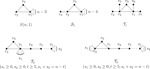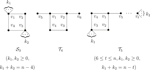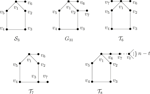Impact Factor 2.638 | CiteScore 2.3
More on impact ›

# Frontiers in Physics## Brief Research Report ARTICLE

Front. Phys., 27 October 2020 | https://doi.org/10.3389/fphy.2020.553261

# Third Smallest Wiener Polarity Index of Unicyclic GraphsWei Fang1,2,Muhan Ma3,FuYuan Chen4,5* andHufeng Dong5
• 1Anhui Province Key Laboratory of Animal Nutritional Regulation and Health, Anhui Science and Technology University, Fengyang, China
• 2School of Mathematical Sciences, Anhui University, Hefei, China
• 3Mathematics and Applied Mathematics, Reading Academy Nanjing University of Information Science and Technology, Nanjing, China
• 4Department of Applied Mathematics, Northwestern Polytechnical University, Xi’an, China
• 5Institute of Statistics and Applied Mathematics, Anhui University of Finance and Economics, Bengbu, China

The Wiener polarity index ${W}_{P}\left(G\right)$ of a graph G is the number of unordered pairs of vertices $\left\{u,v\right\}$ where the distance between u and v is 3. In this paper, we determine the third smallest Wiener polarity index of unicyclic graphs. Moreover, the corresponding extremal graphs are characterized.

## 1. Introduction

Graph theory is one of the most special and unique branches of mathematics. Recently, it has attained much attention among researchers because of its wide range of applications in computer science, electrical networks, interconnected networks, biological networks, chemistry, etc.

The chemical graph theory (CGT) is a fast-growing area among researchers. It helps in understanding the structural properties of a molecular graph. There are many chemical compounds that possess a variety of applications in the fields of commercial, industrial, and pharmaceutical chemistry and daily life and in the laboratory.

In a chemical graph, the vertices represent atoms and edges refer to the chemical bonds in the underlying chemical structure. A topological index is a numerical value that is computed mathematically from the molecular graph. It is associated with the chemical constitution indicating the correlation of the chemical structure with many physical and chemical properties and biological activities .

Let G be a simple and connected graph with $|V\left(G\right)|=n$ and $|E\left(G\right)|=m$. Sometimes we refer to G as a $\left(n,m\right)$ graph. For any $u,v\in V\left(G\right)$, the $distance$${d}_{G}\left(u,v\right)$ between the vertices u and v of G is equal to the length of (number of edges in) the shortest path that connects u and v. ${N}_{G}^{i}\left(u\right)=\left\{v\in V\left(G\right)|{d}_{G}\left(u,v\right)=i\right\}$ is called the ith neighbor vertex set of u. Especially, if $i=1$, then ${N}_{G}^{1}\left(u\right)$(or ${N}_{G}\left(u\right)$ for short) be the of u, and ${d}_{G}\left(u\right)=|{N}_{G}\left(u\right)|$ is called the $degree$ of G. If ${d}_{G}\left(u\right)=1$, then we call u a of G.

A unicyclic graph of order n is a connected graph with n vertices and m edges. It is well-known that every unicyclic graph has exactly one cycle. Let ${\mathcal{U}}_{n}$ denote the class of unicyclic graphs on n vertices. As usual, let ${K}_{1,n-1}$, ${C}_{n}$, and ${P}_{n}$ be the star, cycle, and path of order n, respectively.

Let $\gamma \left(G,k\right)$ denote the number of unordered vertices pairs of G, each of whose distance is equal to k. The Wiener polarity index, denoted by ${W}_{P}\left(G\right)$, is defined to be the number of unordered vertices pairs of distance 3, i.e., ${W}_{P}\left(G\right)=\gamma \left(G,3\right)$.

There is another important graph-based structure descriptor, called Wiener index, based on distances in a graph. The $W\left(G\right)$ is denoted by 

$W(G)=12∑u,v∈V(G)d(u,v)=∑k≥1γ(G,k).$

The name Wiener polarity index is introduced by Harold Wiener  in 1947. In Ref. , Wiener used a linear formula of $W\left(G\right)$ and ${W}_{P}\left(G\right)$ to calculate the boiling points ${t}_{B}$ of the paraffins, i.e.,

$tB=aW(G)+bWP(G)+c,$

where $a,b,$ and c are constants for a given isomeric group.

If ${G}_{1},\cdots ,{G}_{t}$ are the connected components of a graph G, then ${W}_{P}\left(G\right)={\sum }_{i=1}^{t}{W}_{P}\left({G}_{i}\right)$. Therefore, it will suffice to consider the Wiener polarity index of connected graphs.

In 1998, Lukovits and Linert  demonstrated quantitative structure-property relationships in a series of acyclic and cycle-containing hydrocarbons by using the Wiener polarity index. In 2002, Hosoya  found a physicochemical interpretation of ${W}_{P}\left(G\right)$. Du et al.  obtained the smallest and largest Wiener polarity indices together with the corresponding graphs among all trees on n vertices, respectively. Deng  characterized the extremal Wiener polarity indices among all chemical trees of order n. Hou  determined the maximum Wiener polarity index of unicyclic graphs and characterized the corresponding extremal graphs. Lei  determined the extremal trees with the given degree sequence with respect to the Wiener polarity index. In a previous study , the authors obtained the first and second smallest Wiener polarity indexes of unicyclic graphs. In this paper, we determine the third smallest Wiener polarity index of unicyclic graphs. Moreover, all the corresponding extremal graphs are characterized.

## 2. The Third Smallest Wiener Polarity Index of Unicyclic Graphs

The girth $g\left(G\right)$ of a connected graph G is the length of a shortest cycle in G. Let $S\left(n,1\right)$ be the unicyclic graph obtained from ${K}_{1,n-1}$ by adding one edge to two pendant vertices of ${K}_{1,n-1}$.

A of G is a vertex of G which is not a pendant vertex. Suppose U is a unicyclic graph with unique cycle ${C}_{t}$, in the sequel, we agree that $V\left({C}_{t}\right)=\left\{{v}_{1},{v}_{2},\dots ,{v}_{t}\right\}$ and $E\left({C}_{t}\right)=\left\{{v}_{1}{v}_{2},{v}_{2}{v}_{3},\dots ,{v}_{t-1}{v}_{t},{v}_{1}{v}_{t}\right\}$. For $1\le i\le t$, let ${l}_{i}=\text{max}\left\{d\left({v}_{i},x\right)$, where w is a non-pendant vertex and there is exactly one path connecting ${v}_{i}$ with x}.

Lemma 2.1.  Let $U\in {\mathcal{U}}_{n}$, then ${W}_{P}\left(G\right)\ge 0$, where equality holds if and only if $\left(S\left(n,1\right)$ is shown in Figure 1).

FIGURE 1FIGURE 1. Graphs $S\left(n,1\right)$,${\mathcal{S}}_{1}$,${\mathcal{T}}_{1}$, ${\mathcal{T}}_{2}$, and ${\mathcal{T}}_{3}$.

Lemma 2.2. Let $G\in {\mathcal{U}}_{n}$ and $|{N}_{G}^{2}\left(u\right)|\ge k$ for any $u\in V\left(G\right)$. $G+w$ be the new graph obtained from G by adding one vertex w and one edge adjacent to u in G. Then, ${W}_{P}\left(G+w\right)\ge {W}_{P}\left(G\right)+k.$

Proof. Since ${N}_{G+w}\left(w\right)=\left\{u\right\}$ and $|{N}_{G}^{2}\left(u\right)|\ge k$, then ${W}_{P}\left(G+w\right)={W}_{P}\left(G\right)+|{N}_{G}^{3}\left(w\right)|={W}_{P}\left(G\right)+|{N}_{G}^{2}\left(u\right)|\ge {W}_{P}\left(G\right)+k.$

Lemma 2.3 . Suppose $U\in {\mathcal{U}}_{n}\$$\left\{S\left(n,1\right)\right\}$. If $g\left(U\right)=3$ and $n\ge 5$, then ${W}_{P}\left(U\right)\ge n-4$, where equality holds if and only if $U\cong {\mathcal{S}}_{1}$ (${\mathcal{S}}_{1}$ is shown in Figure 1).

Lemma 2.4. Let $U\in {\mathcal{U}}_{n}$. If $g\left(U\right)=3$, then the third smallest Wiener polarity index ${W}_{P}\left(U\right)=n-3$, the equality holds if and only if $U\cong {\mathcal{T}}_{i},1\le i\le 3$$\left({\mathcal{T}}_{1},{\mathcal{T}}_{2}$, and ${\mathcal{T}}_{3}$ are shown in Figure 1$\right)$.

Proof. Let ${C}_{3}=\left\{{v}_{1},{v}_{2},{v}_{3}\right\}$; we consider the next cases.

Case 1. $\text{max}\left\{{l}_{1},{l}_{2},{l}_{3}\right\}=0.$

This implies that U is a unicyclic graph obtained by attaching ${k}_{i}\ge 0$ pendant vertices to ${v}_{i}$, where $1\le i\le 3$. Without loss of generality, let ${k}_{1}+{k}_{3}\ge {k}_{2}$. The graph ${G}_{1i}\left(1\le i\le 4\right)$ is shown in Figure 2; by the definition of Wiener polarity index, we have

$WP(G11)=k1k2+k2k3+k1k3;WP(G12)=k1k2+k2k3;WP(G13)=(k1+k3+1)(k2−1)=k1k2+k2k3−(k1+k3+1−k2);WP(G14)=2(n−5)≥n−4(n≥6);WP(S1)=n−4(n≥5).$
FIGURE 2

Obviously, ${W}_{P}\left({G}_{11}\right)\ge {W}_{P}\left({G}_{12}\right)>{W}_{P}\left({G}_{13}\right);$ the equality holds if and only if ${G}_{11}\cong {G}_{12}$. Then the third smallest Wiener polarity index is ${W}_{P}\left({\mathcal{T}}_{1}\right)=4=n-3$.

Case 2. $\text{max}\left\{{l}_{1},{l}_{2},{l}_{3}\right\}\ge 1.$${G}_{15}$ is the subgraph of U and ${W}_{P}\left({G}_{15}\right)=2$,$|{N}_{{G}_{15}}^{2}\left(u\right)|\ge 1$, the equality holds if and only if $u\ne {v}_{4}$ by Lemma 2.2; we have

$WP(U)≥WP(G15)+n−5=n−3,$

the equality holds if and only if ${\mathcal{T}}_{2}$ or ${\mathcal{T}}_{3}$.

By combining the above arguments, the result follows.

Lemma 2.5 Let$U\in {\mathcal{U}}_{n}$. If$g\left(U\right)=4$, then the third smallest Wiener polarity index${W}_{P}\left(U\right)=n-3$, the equality holds if and only if $U\cong {\mathcal{T}}_{4}$or${\mathcal{T}}_{5}$$\left({\mathcal{T}}_{4}$and${\mathcal{T}}_{5}$are shown inFigure 3).

FIGURE 3FIGURE 3. Graphs ${\mathcal{S}}_{2}$, ${\mathcal{T}}_{4}$, and ${\mathcal{T}}_{5}$.

Proof. Let ${C}_{4}=\left\{{v}_{1},{v}_{2},{v}_{3},{v}_{4}\right\}$, we consider the next cases.

Case 1. $\text{max}\left\{{l}_{1},{l}_{2},{l}_{3},{l}_{4}\right\}=0.$

This implies that U is a unicyclic graph obtained by attaching ${k}_{i}\ge 0$ pendant vertices to ${v}_{i}$, where $1\le i\le 4$. Without loss of generality, let ${k}_{1}+{k}_{3}\ge {k}_{2}+{k}_{4}$. The graph ${G}_{2i}\left(1\le i\le 4\right)$ is shown in Figure 4; by the definition of Wiener polarity index, we have

$WP(G21)=k1k2+k2k3+k3k4+k1k4+∑i=14ki,WP(G22)=k1k2+k2k3+k3k4+k1k4+∑i=14ki,WP(G23)=k1k2+k2k3+k3k4+k1k4+∑i=14ki,WP(G24)=(k1+k3+1)(k2+k4−1)+∑i=14ki.$

Obviously, ${W}_{P}\left({G}_{21}\right)={W}_{P}\left({G}_{22}\right)={W}_{P}\left({G}_{23}\right)>{W}_{P}\left({G}_{24}\right)\ge n-3$; the equality holds if and only if ${G}_{24}\cong {\mathcal{T}}_{4}$. Then the third smallest Wiener polarity index is ${W}_{P}\left({\mathcal{T}}_{4}\right)=3=n-3$.

FIGURE 4

Case 2. $\text{max}\left\{{l}_{1},{l}_{2},{l}_{3},{v}_{4}\right\}\ge 1.$

${\mathcal{S}}_{2}\left({k}_{1}=1$ and ${k}_{2}=0\right)$ is the subgraph of U and ${W}_{P}\left({\mathcal{S}}_{2}\right)=1\left({k}_{1}=1$ and ${k}_{2}=0\right)$, by Lemma 2.2, we have

$WP(U)≥1+n−5=n−4,$

the equality holds if and only if $U\cong {\mathcal{S}}_{2}\left({k}_{1}=1,{k}_{2}=1\right)$. If ${\mathcal{S}}_{2}\left({k}_{1}=1,{k}_{2}=1\right)$ is the induced subgraph of U, by Lemma 2.2, we have

$WP(U)≥2+n−5=n−3,$

the equality holds if and only if $U\cong {\mathcal{T}}_{5}$.

By combining the above arguments, the result follows.

Lemma 2.6 Let $U\in {\mathcal{U}}_{n}$. If $g\left(U\right)=5$, then the third smallest Wiener polarity index ${W}_{P}\left(U\right)=n-3$, the equality holds if and only if $U\cong {\mathcal{T}}_{i},$ $\left(i=6,7,8\right)$ $\left({\mathcal{T}}_{6}$, ${\mathcal{T}}_{7}$, and ${\mathcal{T}}_{8}$are shown inFigure 5).

FIGURE 5FIGURE 5. Graphs ${\mathcal{S}}_{3}$,${G}_{31}$,${\mathcal{T}}_{6}$,${\mathcal{T}}_{7}$, and ${\mathcal{T}}_{8}$.

Proof. Let ${C}_{5}=\left\{{v}_{1},{v}_{2},{v}_{3},{v}_{4},{v}_{5}\right\}$, we consider the next cases.

Case 1. $\text{max}\left\{{l}_{1},{l}_{2},{l}_{3},{l}_{4},{l}_{5}\right\}=0.$

This implies that U is a unicyclic graph obtained by attaching ${k}_{i}\ge 0$ pendant vertices to ${v}_{i}$, where $1\le i\le 5$.

If $n=5$, then there exists only one graph ${C}_{5}$ and ${W}_{P}\left({C}_{5}\right)=0$.

If $n=6$, then there exists only one graph ${\mathcal{S}}_{3}$ and ${W}_{P}\left({\mathcal{S}}_{3}\right)=2=n-4$.

If $n=7$, then there exists three graphs ${G}_{31}$, ${\mathcal{T}}_{6}$, and ${\mathcal{T}}_{7}$, ${W}_{P}\left({G}_{31}\right)=5=n-2$, ${W}_{P}\left({\mathcal{T}}_{6}\right)={W}_{P}\left({\mathcal{T}}_{7}\right)=4=n-3$.

If $n>7$, then ${G}_{31}$ or ${\mathcal{T}}_{6}$ or ${\mathcal{T}}_{7}$ is the subgraph of U and $\text{min}\left\{|{N}_{{G}_{31}}^{2}\left(u\right)|,|{N}_{{\mathcal{T}}_{6}}^{2}\left(u\right)|,|{N}_{{\mathcal{T}}_{7}}^{2}\left(u\right)|\right\}\ge 2$. By Lemma 2.2, we have ${W}_{P}\left(U\right)\ge 4+2+n-8=n-2$.

Case 2. $\text{max}\left\{{l}_{1},{l}_{2},{l}_{3},{l}_{4},{l}_{5}\right\}\ge 1.$${\mathcal{T}}_{8}\left(n=t=7\right)$ is the subgraph of U and ${W}_{P}\left({\mathcal{T}}_{8}\right)=4\left(n=t=7\right)$; meanwhile, $|{N}_{{\mathcal{T}}_{8}}^{2}\left(u\right)|\ge 1$, the equality holds if and only if $u={v}_{7}$. By Lemma 2.2, we have ${W}_{P}\left(U\right)\ge 4+n-7=n-3$, the equality holds if and only if $U={\mathcal{T}}_{8}$.

By combining the above arguments, the result follows.

Lemma 2.7 Let $U\in {\mathcal{U}}_{n}$ and $g\left(U\right)=6$. If $n=6$, then ${W}_{P}\left({C}_{6}\right)=n-3$; if$n>7$, then ${W}_{P}\left(U\right)\ge n-2$.

Proof. When $g\left(U\right)=6$ and $n=6$, then there exists only one graph ${C}_{6}$ and ${W}_{P}\left({C}_{6}\right)=3=n-3$.

When $n\ge 7$, ${C}_{6}$ is the subgraph of U and $|{N}_{{C}_{6}}^{2}\left(u\right)|\ge 2$, by Lemma 2.2, we have ${W}_{P}\left(U\right)\ge 3+2+n-7=n-2$.

Lemma 2.8 Let $U\in {\mathcal{U}}_{n}$, if $g\left(U\right)=s\ge 7$, then ${W}_{P}\left(U\right)\ge n$, the equality holds if and only if $U\cong {C}_{s}$.

Proof. If $U\cong {C}_{s}$, then by the definition of Wiener polarity index, we have ${W}_{P}\left(U\right)=n$.

If $U\mathrm{\ncong }{C}_{s}$, then ${C}_{s}\left(s\ge 7\right)$ is the subgraph of U and $|{N}_{{C}_{s}}^{2}\left(u\right)|\ge 2$. By Lemma 2.2, we have ${W}_{P}\left(U\right)\ge {W}_{P}\left({C}_{s}\right)+2+\left(n-s-1\right)=n+1$.

By combining the above arguments, the result follows.

Theorem 2.9. Let $U\in {\mathcal{U}}_{n}$; then the third smallest Wiener polarity index ${W}_{P}\left(U\right)=n-3$, the equality holds if and only if $U\cong {C}_{6}$ or ${\mathcal{T}}_{i},1\le i\le 8$ $\left({\mathcal{T}}_{1}$,${\mathcal{T}}_{2}$, and ${\mathcal{T}}_{3}$ are shown in Figure 1; ${\mathcal{T}}_{4}$ and ${\mathcal{T}}_{5}$ are shown in Figure 3; ${\mathcal{T}}_{6}$,${\mathcal{T}}_{7}$, and ${\mathcal{T}}_{8}$ are shown in Figure 5$\right)$.

Proof. By Lemma 2.4–2.8, the result follows.

## 3. Conclusions

Chemical graph theory is an important area of research in mathematical chemistry which deals with topology of molecular structure such as the mathematical study of isomerism and the development of topological descriptors or indices. In this paper, we first introduce some useful graph transformations and determine the third smallest Wiener polarity index of unicyclic graphs. In addition, all the corresponding extremal graphs are characterized.

## Data Availability Statement

All datasets presented in this study are included in the article.

## Author Contributions

WF performed conceptualization. FC and HD were responsible for methodology. WF and MM wrote the original manuscript. WF and FC reviewed and edited the article.

## Funding

This work was supported by open project of Anhui University (No. KF2019A01), Natural Science Research Foundation of Department of Education of Anhui Province (No. KJ2019A0817, No. KJ2020A0061), and National Science Foundation of China under grant (No. 11601001).

## Conflict of Interest

The authors declare that the research was conducted in the absence of any commercial or financial relationships that could be construed as a potential conflict of interest.

## References

1. Liu J-B, Wang C, Wang S, Wei B. Zagreb indices and multiplicative zagreb indices of eulerian graphs. Bull. Malays. Math. Sci. Soc (2019) 42:67–78. doi:10.1007/s40840-017-0463-2

2. Liu J-B, Pan X-F, Hu F-T, Hu F-F. Asymptotic Laplacian-energy-like invariant of lattices. Appl Math Comput (2015) 253:205–14. doi:10.1016/j.amc.2014.12.035

3. Liu J-B, Pan X-F. Minimizing Kirchhoff index among graphs with a given vertex bipartiteness. Appl Math Comput (2016) 291:84–8. doi:10.1016/j.amc.2016.06.017

4. Wiener H. Structural determination of paraffin boiling points. J Am Chem Soc (1947) 69:17–20. doi:10.1021/ja01193a005

5. Lukovits I, Linert W. Polarity-numbers of cycle-containing structures. J Chem Inf Comput Sci (1998) 38:715–9. doi:10.1021/ci970122j

6. Hosoya H. Mathematical and chemical analysis of Wieners polarity number. In: Rouvray DH, King RB, editors Topology in chemistry-discrete mathematics of molecules. Vol. 57. Chichester: Horwood (2002).

7. Du W, Li X, Shi Y. Algorithms and extremal problem on Wiener polarity index. MATCH Commun. Math. Comput. Chem (2009) 62:235–44.

8. Lei H, Li T, Shi Y, Wang H. Wiener polarity index and its generalization in trees. MATCH Commun. Math. Comput. Chem (2017) 78:199–212.

9. Hou H, Liu B, Huang Y. The maximum Wiener polarity index of unicyclic graphs. Appl Math Comput (2011) 218:10149–57. doi:10.1016/j.amc.2012.03.090

10. Liu M, Liu B. On the wiener polarity index. MATCH Commum. Math. Comput. Chem (2011) 66:293–304.

Keywords: wiener polarity index, minimum, unicyclic graph, extremal graph, electrical networks

Citation: Fang W, Ma M, Chen F and Dong H (2020) Third Smallest Wiener Polarity Index of Unicyclic Graphs. Front. Phys. 8:553261. doi: 10.3389/fphy.2020.553261

Received: 18 April 2020; Accepted: 09 September 2020;
Published: 27 October 2020.

Edited by:

Muhammad Javaid, University of Management and Technology, Pakistan

Reviewed by:

YaJing Wang, North University of China, China
Akbar Ali, University of Hail, Saudi Arabia

Copyright © 2020 Fang, Ma, Chen and Dong. This is an open-access article distributed under the terms of the Creative Commons Attribution License (CC BY). The use, distribution or reproduction in other forums is permitted, provided the original author(s) and the copyright owner(s) are credited and that the original publication in this journal is cited, in accordance with accepted academic practice. No use, distribution or reproduction is permitted which does not comply with these terms.

*Correspondence: Fuyuan Chen, chen_fuyuan@sina.com